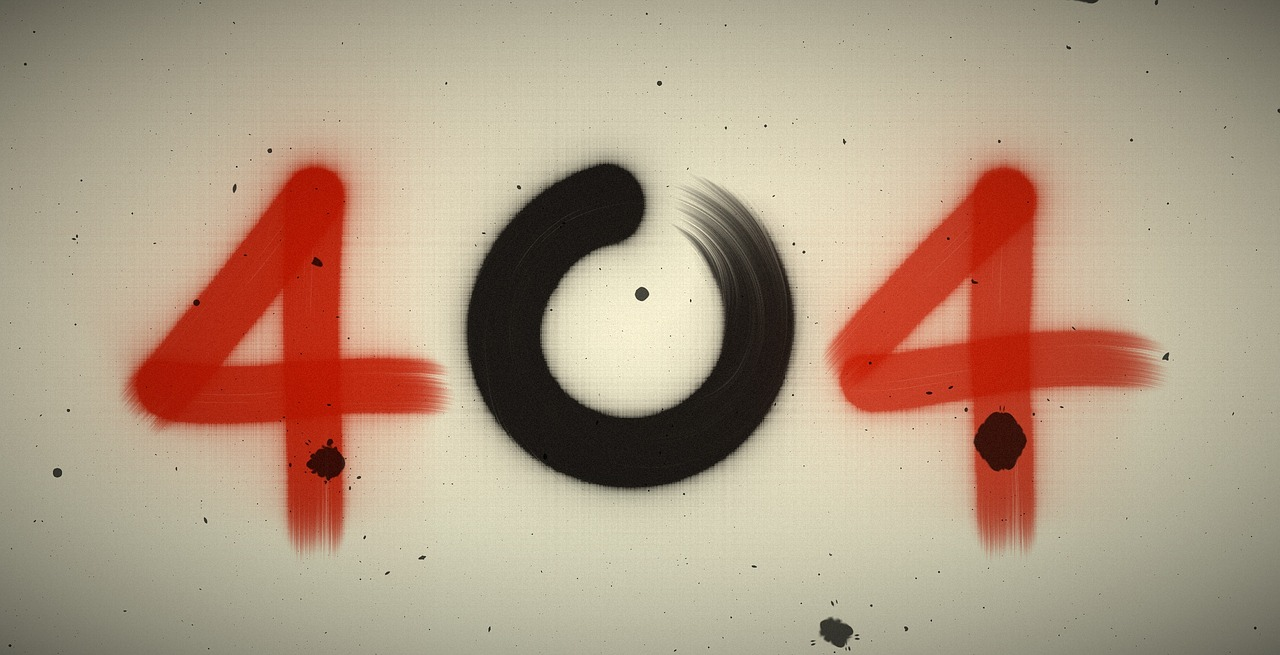## Post per Page

Suppose you are reading a really long text in a bid to understand something. What happens? Slowly, you start feeling dull and bored. Your eyes and mind become saturated. Isn’t it? But, if you find some related diagrams or any sort of graphs, flowcharts, or pictures along with the text, do they make any difference? A big yes! You start visualizing the things vividly in front of you and begin to comprehend the things much more clearly. Right?

This is something that most of us can relate to. Undoubtedly, diagrams and other pictorial descriptions steer the simplification of things and make them a piece of cake to feed on. And, Feynman diagrams are a living example of this. Named after the American physicist Richard Feynman, who introduced these diagrams in 1948, the Feynman diagrams are a simple yet elegant visualization of the interaction of subatomic particles that can otherwise be very complex and difficult to understand. In the simplest words, “a Feynman diagram shows what happens when elementary particles collide”.

How do particles interact?

Interactions are the basic reason behind the existence of stars, the existence of life, more aptly, the existence of this whole universe. And, to understand these Feynman diagrams also, it is imperative to know that how do the particle interactions actually take place. As we know, the fermions, which are the elementary particles with half-integral spin, are often referred to as matter particles. A famous example of a fermion is an electron. In contrast, the bosons with integral spin are termed as the force carriers or the field particles. Both these particles are the basis of all the interactions taking place in the universe.

At the quantum level, the fermions interact via emission and absorption of these field particles associated with the fundamental interactions of matter, particularly the photons in case of the electromagnetic force, gluons for the strong force, and W and Z bosons as far the weak force is concerned. So, the crux of the story is that whenever two particles interact, some other particles get exchanged between them. And Feynman diagrams come in handy in having a 2-dimensional picture of these interactions happening in space-time.

Symbolic representations used in Feynman diagrams:

As already mentioned above, these are basically the 2D diagrams in space-time. But, whenever we draw any diagram or anything like that, we have to take care of some steps, rules, and notations. And, the Feynman diagrams are no different. There are several rules that we need to obey while drawing them and these are as mentioned below (also refer to the image given below as you read the rules):

First of all, the axes need to be defined. One of the two axes is taken to represent the direction of space, while the other one acts as the axis of time.

Different types of lines are assigned to particles belonging to different categories. Where straight lines depict fermions like electrons, the bosons such as photons are represented with wavy/curly lines.

The point where any three lines meet is termed as a Vertex. So, practically speaking, a vertex is that point where interaction actually occurs, and a particle gets either absorbed or emitted.

Apart from this, the incoming particle is shown with an arrowhead pointing towards the vertex, whereas the arrowhead for the outgoing particle points away from the vertex.

All the above-mentioned notations are for ordinary particles. But, what about the antiparticles? Aren’t Feynman diagrams applicable to them? Well, these diagrams hold for antiparticles as much as they do for normal particles. The only difference is that antiparticles are represented as ordinary matter particles moving backward in time. In other words, the direction of the arrowhead is reversed for them. An incoming antiparticle has its arrowhead pointed away from the vertex, while the outgoing one has it pointed towards it.

The image below shows a Feynman diagram including both particles and antiparticles. It shows the annihilation of an electron (e−) by a positron (e+). This annihilation of the particle-antiparticle pair leads to forming a muon (μ−) and an antimuon (μ+). Both antiparticles are shown moving backward in time while the particles are moving forward.

Why are Feynman diagrams so useful?

These diagrams are one of the fundamental tools used to make precise calculations for the probability of occurrence of any process by physicists. Different diagrams can represent a single interaction process, and the contribution from each diagram is taken into consideration while calculating this probability. However, the mathematical expressions involved in calculating these probabilities are quite complex, a lot simpler as compared to other techniques!

Although the American theoretical physicist Richard Feynman first introduced these diagrams only as a bookkeeping device for simplifying lengthy calculations in the area of quantum electrodynamics, these diagrams have come a long way now. Even David Kaiser once quoted, “Since the middle of the 20th century, theoretical physicists have increasingly turned to this tool to help them undertake critical calculations. Feynman diagrams have revolutionized nearly every aspect of theoretical physics.” Undoubtedly, these diagrams are one of Feynman’s finest contributions ever made to the Physics fraternity!